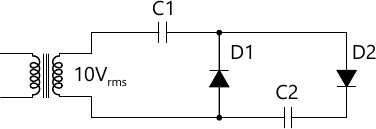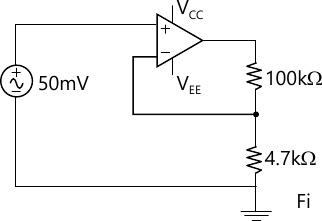MORE IN Basic Electronics Engineering (BXE)
SPPU First Year Engineering (Semester 1)
Basic Electronics Engineering (BXE)
October 2012
Total marks: --
Total time: --
INSTRUCTIONS
(1) Assume appropriate data and state your reasons
(2) Marks are given to the right of every question
(3) Draw neat diagrams wherever necessary

Answer any one question from Q1 & Q2
1 (a) A diode used as half wave rectifire, whose internal resistance is 20? is to supply power to a 1000? load from a 110Vrms Source of Supply.
Calculate:
6 M
1 (b) (i) Sketch the CE output characteristics and indicate the active, saturation and cut-off regions.
3 M
1 (b) (ii) Sketch a family of CE input characteristics and explain the shape of these curves qualitatively.
3 M

2 (a)

Refer the circuit diagram of Fig. 1
(i) Idenify the circuit.
(ii) Calculate voltage across capacitor C1 and C2.6 M
2 (b) Explain CE transistor as a switch and why CB and CC configurations are not preferred as a switch.
6 M

Answer any one question from Q3 & Q4
3 (a)

Determine the gain and output voltage of the amplifier in fig. 2. Assume ideal Op.-Amp6 M
3 (b) Realize EX-OR gate using NAND only.
3 M
3 (c) Prove the following using Boolean Algebra:
A+ A ?B+A? B=A+B
3 M

4 (a) The timing component in square wave generator circuit using IC555 are RA=10K, RB=20K and C=0.01 ?F, Calculate:
(i) Frequency of Oscillation
(ii) Percentage Duty Cycle
6 M
4 (b) Draw a full adder circuit using two half adders. Use :
(a) Block diagram of Half Adder (b) Circuit diagram of Half Adder
6 M

Answer any one question from Q5 & Q6
5 (a) Explain the construction, working and characteristics of SCR.
6 M
5 (b) Draw constructional details of LVDT. Explain its operation. State its advantages and disadvantages.
7 M

6 (a) Draw block diagram of Electronics Weighing Machine and explain its operation.
6 M
6 (b) Explain the construction, working and characteristics of TRAIC.
7 M

Answer any one question from Q7 & Q8
7 (a) Draw and explain Electromagnetic Spectrum.
7 M
7 (b) Draw and explain the block diagram of GSM system.
6 M

8 (a) Define Modulation Index and explain the effect of Modulation Index in FM.
6 M
8 (b) Write short note on Twisted Pair Cables and explain problem associated with Twisted Pair Cables.
7 M

More question papers from Basic Electronics Engineering (BXE)